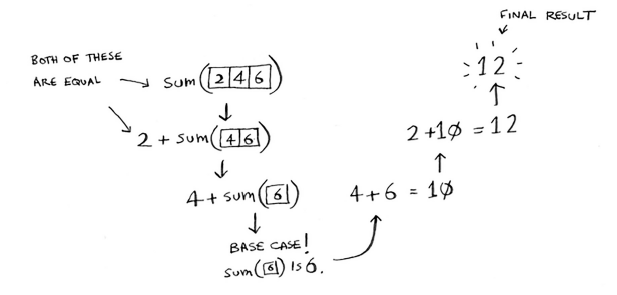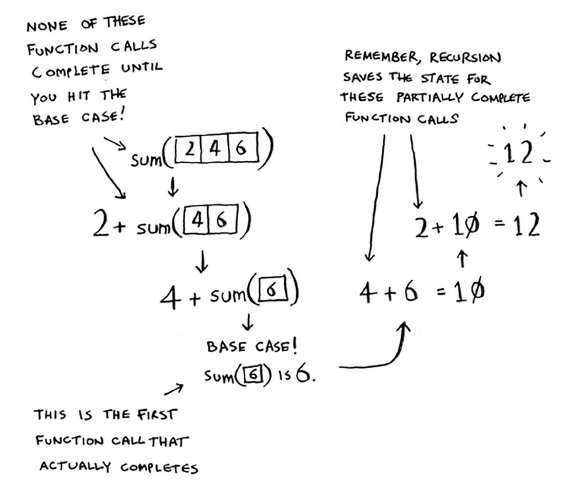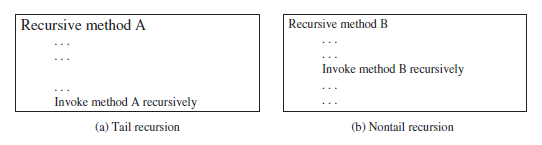Recursion

# Recursion

2020-02-13T23:46:37.002Z

Recursion is when a function calls itself, reducing a task to smaller subtasks until reaching a base subtask that it can perform without calling itself, and then using the result of that base subtask in the smaller subtasks until reaching a final result.

At least one base case is needed to end the recursion—otherwise, the algorithm recurses until it overflows the stack. There are recursive and iterative (non-recursive) algorithms. When you are writing a recursive function involving an array, the base case is often an empty array or an array with one element. In other words, the case in which the method will not recurse is the base case.

Divide-and-conquer algorithms are all recursive algorithms:

1. Figure out a simple case as the base case.
2. Figure out how to reduce your problem and get to the base case.

You need to move closer to the base case with every recursive call.

Recursive countdown:

``````function countdown(number) {
console.log(number);
if (number <= 0) {
// base case
return;
} else {
// recursive case
countdown(number - 1);
}
}

countdown(10);
``````

Recursive sum:

``````function sum(arr) {
if (arr.length === 0) {
// base case
return 0;
} else {
// recursive case
return arr.shift() + sum(arr);
}
}

sum([2, 3, 6]);
``````Recursive sumTracking state in a recursive sum

Recursion is useful for tasks that can be defined in terms of similar subtasks. For example, sort, search, and traversal problems often have simple recursive solutions. A recursive function performs a task in part by calling itself to perform the subtasks. At some point, the function encounters a subtask that it can perform without calling itself. This case, in which the function does not recurse, is called the "base case"; the former, in which the function calls itself to perform a subtask, is referred to as the "recursive case".

Every recursive case must eventually lead to a base case. Every recursive call reduces the original problem, bringing it increasingly closer to a base case until it becomes that case.

## Performance

There is no performance benefit to using recursion; in fact, loops are sometimes better for performance. Each function call takes up some memory, and when your stack is too tall, your computer is saving information for many function calls.

Recursion is powerful, but it is not always the best approach, and rarely is it the most efficient approach. This is due to the relatively large overhead for function calls on most platforms. For a simple recursive function, most computer architectures spend more time on call overhead than on the actual calculation. Iterative functions, which use looping constructs instead of recursive function calls, do not suffer from this overhead and are frequently more efficient.

Iterative solutions are usually more efficient than recursive solutions.

Any problem that can be solved recursively can be solved nonrecursively with iterations. Recursion has some negative aspects: it uses up too much time and too much memory. Why, then, should you use it? In some cases, using recursion enables you to specify a clear, simple solution for an inherently recursive problem that would otherwise be difficult to obtain. Examples are the directory-size problem, the Tower of Hanoi problem, and the fractal problem, which are rather difficult to solve without using recursion.

## Tail recursion

In head recursion, the recursive call, when it happens, comes before other processing in the function (think of it happening at the top, or head, of the function). In tail recursion, it's the opposite—the processing occurs before the recursive call; a tail-recursive method has no pending operations after a recursive call.Tail recursion

Tail recursion is desirable: because the method ends when the last recursive call ends, there is no need to store the intermediate calls in the stack. Compilers can optimize tail recursion to reduce stack size.